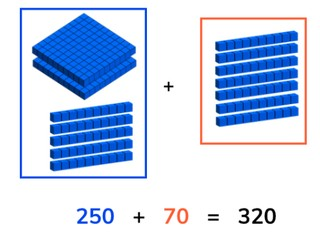Addition to 1,000 with multiples of ten

# Addition to 1,000 with multiples of ten

Students learn to add numbers up to 1,000 with multiples of ten crossing hundred

No account needed.8,000 schools use Gynzy92,000 teachers use Gynzy1,600,000 students use Gynzy

## General

Students practicing adding numbers up to 1,000 and focus on using multiples of ten. They also cross hundreds when adding the two numbers.

## Standards

CCSS.Math.Content.2.NBT.B.7

## Learning objective

Students will be able to add numbers up to 1,000 with multiples of ten crossing hundreds.

## Introduction

Ask a student at random to come to the front of the class and drag the MAB blocks to the table on the interactive whiteboard. Then have a second student drag the next given number of MAB blocks to the table on the interactive whiteboard. Discuss the math problem that has been created by the two sets of MAB blocks.

## Development

Check that students are able to add numbers to 1,000 with multiples of ten crossing hundreds by asking the following questions:
- Why is it useful to be able to add numbers to 1,000?
- How do you solve 260 + 90, and 350 + 170?

## Guided Practice

Students practice by adding two numbers to 1,000 with visual support. Then they are only given the problem and asked to solve. Finally students are given story problems to solve.

## Closing

Remind students that it is important to be able to add using multiples of ten, because you can then easily find the total. Show the money on the interactive whiteboard and ask students which addition problem matches the image and what the total is. Then ask students to solve the abstract math problem, and end with a story problem. Ask students to explain their strategy for solving the problems. To close, have students create math problems in pairs. Ask each student to write a number less than 500 on a sheet of paper. Then have them find a partner, and solve the addition problem their two numbers create together. You can repeat this a number of times, with students finding new partners and adding their numbers together.

## Teaching tips

If students have difficulty adding multiples of ten, you can support their addition by making use of MAB blocks, other manipulatives, or a number line. Remind students that they can add in different jumps, including jumps of 1, 2, 5, 10, 50, and 100. Help students by starting with an easier problem that crosses hundreds like 150 + 60, and then increase the difficulty as they become more comfortable with the addition process.

## Instruction materials

Paper for students to write their number for the closing of the lesson.

### The online teaching platform for interactive whiteboards and displays in schools

• Save time building lessons

• Manage the classroom more efficiently

• Increase student engagement